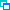# Fibonacci Sequence Visualisor

Designed for Casey Mongoven as a tool for his music composition, this page will calculate large numbers of terms of various sequences that are related to the Fibonacci Numbers and display them in a graph-like form so that they are easily visualised. Casey uses this tool in the creation of his musical scores.

Select which part of the sequence A(n) you want with the From and To boxes giving the starting and ending values of n. The series numbers are shown in the Sequence Values box and in the Sequence Plot they are translated into squares (give the size in pixels) on a "graph" where the direction of the graph's base line is selected (>=across, v=down, ^=up) and the numbers becomes distances from that base line.

The Spectrum and Signature numbers can be expressions such as sqrt(2) or sin(Pi/3) (trig functions use radian meaure). Phi means (sqrt(5)+1)/2 and phi is (sqrt(5)-1)/2.

Click on a Sequence name button to see the Sequence Plot and Values.

Notes on the sequences themselves and what they mean are lower down on this page.

Sequence A(n): for these values of n: Direction of display
 G(,)
 of Phi, sqrt, trig, +.-.*,/ allowed here
from
to
notes pixels
direction of increase
Sequence Plot:

Sequence values:

Vertical Para-Fib
A(n) is the row number of n in the Wythoff array
Horizontal Para-Fib
A(n) is the column number of n in the Wythoff array
R(n)
is the number of sets of Fibonacci numbers (1,2,3,5,8,13...) that sum to n, e.g. 8 is 8 itself or 3+5 or 1+2+5. These are the Fibonacci representations of 8 and there are 3 of them, so the 8-th number in this series, R(8), is 3. No value is repeated repeated in the sums.
Rabbit Sequence
or the Golden String is a sequence of 0s and 1s with many relationships with the Fibonacci numbers and the golden ratios Phi and phi
G(a,b,n)
is the Generalized Fibonacci Sequence that starts from a and b and subsequent values are the sum of the last two. G(0,1) gives 0,1,1,2,3,5,... which is the Fibonacci sequence; G(2,1) is 2,1,3,4,7,11,... the Lucas Numbers and so on.
Mod m
All of these are increasing sequences of number and interesting visualisation patterns are producing by taking the remainders when divided by m which is called mod m. To see the actual numbers in a G series, use a large molulus such as 1000.
#reps
In the same way that R(n) counts the number of sets of Fibonacci numbers each summing to n, this is the number of different sets of values in the G series that have a sum of 1, 2, 3, ,.. . #reps means the number of representations.
Spectrum of x
.. is the series of the whole number parts of the multiples of x, i.e. { Int(n x) | n=1,2,3,...}. The whole number part is just that part before the decimal point so that the whole number part of 3.872 is 3. e.g. Phi = 1.6180339... so its multiples including itself are
Phi = 1.61803, 2 Phi = 3.23607, 3 Phi = 4.8541, 4 Phi = 6.47214, 5 Phi = 8.09017...
and so its spectrum is
1, 3, 4, 6, 8, ...
Each real number has a unique spectrum. If the number x is not expressible as a fraction (x is irrational), the spectrum is called the Beatty Sequence of x.
More at mathworldThe signature of x
Take all the multiples of the given number, x, and add on to each the whole numbers, c, in turn from c=1 and we get a table of values of c+dx. E.g. if x is Phi=1.618.. we have the table shown here:
c+dPhid
1 2 3 4
c 1 2.62 4.24 5.85 7.47
2 3.62 5.24 6.85 8.47
3 4.62 6.24 7.85 9.47
4 5.62 7.24 8.85 10.47
5 6.62 8.24 9.85 11.47
Each column has numbers with the same decimal part since we are just adding whole numbers to that particular multiple of Phi that is indicated by the column header (y). If x is irrational then no exact values will ever be repeated in the table.
We now sort the entries in the table into increasing order. The row values (c) of each entry in this ordered list is called the signature of x.
Sorting, with (c,d) being the row and column numbers, we have 2.62 at (1,1), 3.62 at (2,1), 4.24 at (1,2), 4.62 at (3,1), 5.24 at (2,2) and so on. The sequence of row numbers (c) in this list is the signature of Phi: 1,2,1,3,2,...
More at Mathworld.© Dr Ron Knott for Casey Mongoven: version 7 February 2005